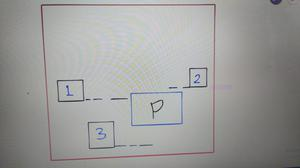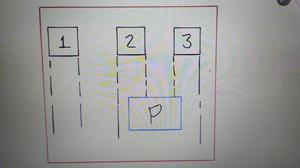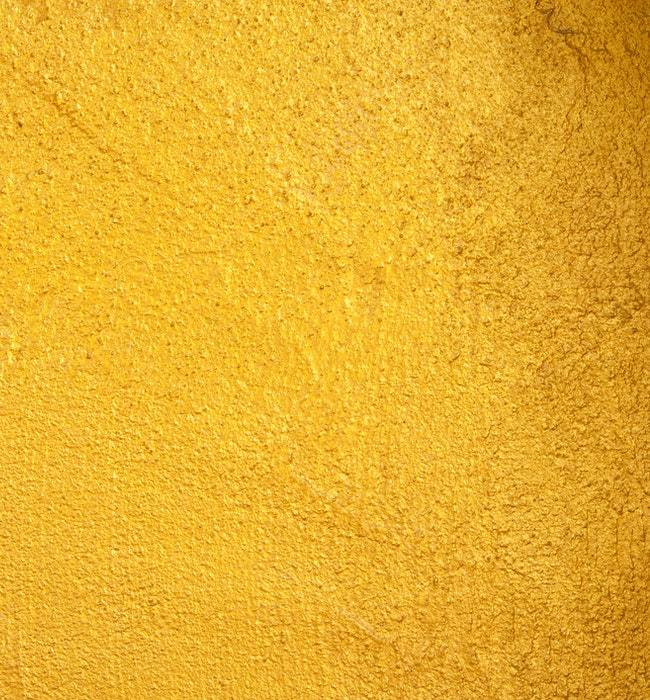# Collision Detection in PyGame

Prerequisite: Introduction to pygame

Collision detection is a very often concept and used in almost games such as ping pong games, space invaders, etc. The simple and straight forward concept is to match up the coordinates of the two objects and set a condition for the happening of collision.

In this article, we will be detecting a collision between two objects where one object would be coming in a downward direction and the other one would be moved from the left and right with key control. It’s the same as to escape from the block falling on the player and if block collides the player, then the collision is detected.

Let’s see the part wise implementation:

Part 1:

## Python3

 `# import required libraries ` `import` `pygame ` `import` `random ` ` `  `# initialize pygame objects ` `pygame.init() ` ` `  `# define the colours ` `white ``=` `(``255``, ``255``, ``255``) ` `red ``=` `(``255``, ``0``, ``0``) ` `green ``=` `(``0``, ``255``, ``0``) ` `blue ``=` `(``0``, ``0``, ``255``) ` `black ``=` `(``0``, ``0``, ``0``) ` ` `  `# set the Dimensions ` `width ``=` `650` `height ``=` `700` ` `  `# size of a block ` `pixel ``=` `64` ` `  `# set Screen ` `screen ``=` `pygame.display.set_mode((width,  ` `                                  ``height)) ` ` `  `# set caption ` `pygame.display.set_caption(``"CORONA SCARPER"``) ` ` `  `# load the image ` `gameIcon ``=` `pygame.image.load(``"rectangleBlock.png"``) ` ` `  `# set icon ` `pygame.display.set_icon(gameIcon) ` ` `  `# load the image ` `backgroundImg ``=` `pygame.image.load(``"wallBackground.jpg"``)`

This is the basic simple code for creating a window screen and setting up the caption, icon, and some pre-defined variables which are not so important to get into in deep. The pixel variable is the size of the block image i.e 64 pixels.

Part 2:

## Python3

 `# load the image ` `playerImage ``=` `pygame.image.load(``"player.png"``) ` ` `  `# set the position ` `playerXPosition ``=` `(width``/``2``) ``-` `(pixel``/``2``) ` ` `  `# So that the player will be  ` `# at height of 20 above the base ` `playerYPosition ``=` `height ``-` `pixel ``-` `10`      ` `  `# set initially 0 ` `playerXPositionChange ``=` `0` ` `  `# define a function for setting ` `# the image at particular ` `# coordinates ` `def` `player(x, y): ` `  ``# paste image on screen object ` `  ``screen.blit(playerImage, (x, y)) ` ` `  `# load the image ` `blockImage ``=` `pygame.image.load(``"rectangleBlock.png"``) ` ` `  `# set the random position ` `blockXPosition ``=` `random.randint(``0``, ` `                                ``(width ``-` `pixel)) ` ` `  `blockYPosition ``=` `0` `-` `pixel ` ` `  `# set the speed of ` `# the block ` `blockXPositionChange ``=` `0` `blockYPositionChange ``=` `2`     ` `  `# define a function for setting ` `# the image at particular ` `# coordinates ` `def` `block(x, y): ` `  ``# paste image on screen object ` `  ``screen.blit(blockImage, ` `              ``(x, y))`

Here we are displaying the player and the block at their respective X and Y positions. The block’s X position is random in each round.

Note: Wherever the pixel word is used, it is used to subtract 64 pixels from the given position so that the full image is shown

E.g: The block if shown is at width position, then it will be drawn starting from that point and hence it will be shown out of the screen. Hence we are subtracting 64 pixels to be viewing the image full

Now,Horizontal Collision

First, we check if the block passes through the player’s horizontal line. We will set the range such that the block’s base horizontal line should match the player’s horizontal line. In the above image, block 2 and 3 having their baseline out of range of player P’s top and bottom surface line. Hence, they are not in the collision range. Block 1’s baseline is in the range of the player P’s top and bottom. Hence we further see that the block comes in the range of the player’s vertical range or not.Vertical Collision

Here, we check the range of player’s left and right side surface dimensions with the blocks left and right surfaces. Here, the blocks 2 and 3 when coming down, will collide the player, and hence the range of 2 and 3 block’s range are between player’s X and player’s Y position.

Hence, this concept is to used to detect the collision.

Part 3:

## Python3

 `# define a function for  ` `# collision detection ` `def` `crash(): ` `  ``# take a global variable ` `  ``global` `blockYPosition ` ` `  `  ``# check conditions ` `  ``if` `playerYPosition < (blockYPosition ``+` `pixel): ` ` `  `      ``if` `((playerXPosition > blockXPosition  ` `           ``and` `playerXPosition < (blockXPosition ``+` `pixel)) ` `          ``or` `((playerXPosition ``+` `pixel) > blockXPosition ` `           ``and` `(playerXPosition ``+` `pixel) < (blockXPosition ``+` `pixel))): ` ` `  `          ``blockYPosition ``=` `height ``+` `1000`

the crash function defines the collision condition.

In the first IF condition, we check the horizontal collision. Here, if the player’s Y position is less than blocks Y position, i.e the block is passed away from the player’s horizontal range, then the next condition is to be checked is horizontal. Pixel is added to blockYPosition because its Y position is at top of the block and the bottom or base of the block is a block’s top position + its pixel size(image size).

The second IF condition checks the vertical collision. If the block is passing from the horizontal range then only check for vertical, so that the block’s collision is detected in all its four sides. Now, if the players X position is greater then block’s X position, i.e block is at left w.r.t player. Here, if the block’s starting position is less than player starting position and block’s end position(block Y position + pixel) is greater than player starting position, this means that the block will overlap the player’s starting position and hence collide. This is shown in the above vertical collision image for block 2.

Similarly, the second range is given that if the blocks start position is less than the player’s end position and blocks end position is greater than the player’s end position. This is shown for the same image for block 3.

The image clearly explains the view of the collision.

Hence, if a collision happens, we will move the block to below the screen i.e at 1000+ distance below so that it would be invisible and the new block will not appear.

Part 4:

## Python3

 `running ``=` `True` ` `  `while` `running: ` `  ``# set the image on screen object ` `  ``screen.blit(backgroundImg, (``0``, ``0``)) ` ` `  `  ``# loop through all events ` `  ``for` `event ``in` `pygame.event.get(): ` `        `  `      ``# check the quit condition ` `       ``if` `event.``type` `=``=` `pygame.QUIT: ` `          ``# quit the game ` `          ``pygame.quit() ` ` `  `       ``# movement key control of player ` `       ``if` `event.``type` `=``=` `pygame.KEYDOWN: ` ` `  `          ``if` `event.key ``=``=` `pygame.K_RIGHT: ` ` `  `              ``playerXPositionChange ``=` `3` ` `  `          ``if` `event.key ``=``=` `pygame.K_LEFT: ` ` `  `              ``playerXPositionChange ``=` `-``3` ` `  `       ``if` `event.``type` `=``=` `pygame.KEYUP: ` ` `  `           ``if` `event.key ``=``=` `pygame.K_RIGHT ``or` `pygame.K_LEFT: ` ` `  `               ``playerXPositionChange ``=` `0`

This is the gaming loop where the movement of the player is controlled. and the game is started.

Part 5:

## Python3

 `# Boundaries to the Player ` ` `  `# if it comes at right end, ` `# stay at right end and  ` `# does not exceed ` `if` `playerXPosition >``=` `(width ``-` `pixel): ` `  ``playerXPosition ``=` `(width ``-` `pixel)  ` `   `  `# if it comes at left end,  ` `# stay at left end and ` `# does not exceed ` `if` `playerXPosition <``=` `0``: ` `  ``playerXPosition ``=` `0`

These are the boundaries to the player so that when the player moves to its rightmost or leftmost position on the screen, it should not go further and bounce back.

Part 6:

## Python3

 `# Multiple Blocks Movement after each other ` `# and condition used because of game over function ` `if` `(blockYPosition >``=` `height ``-` `0` `and`  `    ``blockYPosition <``=` `(height ``+` `200``)): ` `   `  `  ``blockYPosition ``=` `0` `-` `pixel ` `   `  `  ``# randomly assign value in range ` `  ``blockXPosition ``=` `random.randint(``0``, (width ``-` `pixel))`

When the block without colliding goes away from the player, then we need to let him come again from the top. Hence we provide a condition that if the block’s Y position is below the height of the screen and below height+200(as above 1000+, the block appears when the block has collided), then move it again at the top.

Part 7:

## Python3

 `# movement of Player ` `playerXPosition ``+``=` `playerXPositionChange ` ` `  `# movement of Block ` `blockYPosition ``+``=` `blockYPositionChange ` ` `  `# player Function Call ` `player(playerXPosition, playerYPosition) ` ` `  `# block Function Call ` `block(blockXPosition, blockYPosition) ` ` `  `# crash function call ` `crash() ` ` `  `# upadte screen ` `pygame.display.update()`

At the last, the movement of the player and the block is given and the screen is refreshed

Output:

My Personal Notes arrow_drop_upCheck out this Author's contributed articles.

If you like GeeksforGeeks and would like to contribute, you can also write an article using contribute.geeksforgeeks.org or mail your article to contribute@geeksforgeeks.org. See your article appearing on the GeeksforGeeks main page and help other Geeks.

Please Improve this article if you find anything incorrect by clicking on the "Improve Article" button below.

Article Tags :

2

Please write to us at contribute@geeksforgeeks.org to report any issue with the above content.